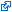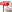PelletierDutilleulLarocqueEtAl2004

Référence

Pelletier, B., Dutilleul, P., Larocque, G., Fyles, J.W. (2004) Fitting the linear model of coregionalization by generalized least squares. Mathematical Geology, 36(3):323-344. (Scopus)

Résumé

In geostatistical studies, the fitting of the linear model of coregionalization (LMC) to direct and cross experimental semivariograms is usually performed with a weighted least-squares (WLS) procedure based on the number of pairs of observations at each lag. So far, no study has investigated the efficiency of other least-squares procedures, such as ordinary least squares (OLS), generalized least squares (GLS), and WLS with other weighing functions, in the context of the LMC. In this article, we compare the statistical properties of the sill estimators obtained with eight least-squares procedures for fitting the LMC: OLS, four WLS, and three GLS. The WLS procedures are based on approximations of the variance of semivariogram estimates at each distance lag. The GLS procedures use a variance-covariance matrix of semivariogram estimates that is (i) estimated using the fourth-order moments with sill estimates (GLS 1), (ii) calculated using the fourth-order moments with the theoretical sills (GLS 2), and (iii) based on an approximation using the correlation between semivariogram estimates in the case of spatial independence of the observations (GLS 3). The current algorithm for fitting the LMC by WLS while ensuring the positive semidefiniteness of sill matrix estimates is modified to include any least-squares procedure. A Monte Carlo study is performed for 16 scenarios corresponding to different combinations of the number of variables, number of spatial structures, values of ranges, and scale dependence of the correlations among variables. Simulation results show that the mean square error is accounted for mostly by the variance of the sill estimators instead of their squared bias. Overall, the estimated GLS 1 and theoretical GLS 2 are the most efficient, followed by the WLS procedure that is based on the number of pairs of observations and the average distance at each lag. On that basis, GLS 1 can be recommended for future studies using the LMC. © 2004 International Association for Mathematical Geology.

Format EndNote

Vous pouvez importer cette référence dans EndNote.

Format BibTeX-CSV

Vous pouvez importer cette référence en format BibTeX-CSV.

Format BibTeX

Vous pouvez copier l'entrée BibTeX de cette référence ci-bas, ou l'importer directement dans un logiciel tel que JabRef.

@ARTICLE { PelletierDutilleulLarocqueEtAl2004,
AUTHOR = { Pelletier, B. and Dutilleul, P. and Larocque, G. and Fyles, J.W. },
TITLE = { Fitting the linear model of coregionalization by generalized least squares },
JOURNAL = { Mathematical Geology },
YEAR = { 2004 },
VOLUME = { 36 },
PAGES = { 323-344 },
NUMBER = { 3 },
NOTE = { cited By 29 },
ABSTRACT = { In geostatistical studies, the fitting of the linear model of coregionalization (LMC) to direct and cross experimental semivariograms is usually performed with a weighted least-squares (WLS) procedure based on the number of pairs of observations at each lag. So far, no study has investigated the efficiency of other least-squares procedures, such as ordinary least squares (OLS), generalized least squares (GLS), and WLS with other weighing functions, in the context of the LMC. In this article, we compare the statistical properties of the sill estimators obtained with eight least-squares procedures for fitting the LMC: OLS, four WLS, and three GLS. The WLS procedures are based on approximations of the variance of semivariogram estimates at each distance lag. The GLS procedures use a variance-covariance matrix of semivariogram estimates that is (i) estimated using the fourth-order moments with sill estimates (GLS 1), (ii) calculated using the fourth-order moments with the theoretical sills (GLS 2), and (iii) based on an approximation using the correlation between semivariogram estimates in the case of spatial independence of the observations (GLS 3). The current algorithm for fitting the LMC by WLS while ensuring the positive semidefiniteness of sill matrix estimates is modified to include any least-squares procedure. A Monte Carlo study is performed for 16 scenarios corresponding to different combinations of the number of variables, number of spatial structures, values of ranges, and scale dependence of the correlations among variables. Simulation results show that the mean square error is accounted for mostly by the variance of the sill estimators instead of their squared bias. Overall, the estimated GLS 1 and theoretical GLS 2 are the most efficient, followed by the WLS procedure that is based on the number of pairs of observations and the average distance at each lag. On that basis, GLS 1 can be recommended for future studies using the LMC. © 2004 International Association for Mathematical Geology. },
AUTHOR_KEYWORDS = { Direct and cross semivariograms; Empirical variance and bias; Fourth-order moments; Multivariate nested semivariogram model; Positive semidefiniteness; Sill estimators },
DOCUMENT_TYPE = { Article },
DOI = { 10.1023/B:MATG.0000028440.29965.2d },
ISSN = { 08828121 },
KEYWORDS = { geostatistics; least squares method; mathematical analysis },
SOURCE = { Scopus },
URL = { http://www.scopus.com/inward/record.url?eid=2-s2.0-3042803149&partnerID=40&md5=9a69c7c0cb328c2a9bc30d37a88d3a15 },
}

Abonnez-vous à
l'Infolettre du CEF!

********************************************************** ***************** Pub - Mycorhizes_2019 ****************** **********************************************************

********************************************************** ***************** Pub - Symphonies_Boreales ****************** **********************************************************

********************************************************** ***************** Boîte à trucs *************** **********************************************************

CEF-Référence
La référence vedette !

Jérémie Alluard (2016) Les statistiques au moments de la rédaction• Ce document a pour but de guider les étudiants à intégrer de manière appropriée une analyse statistique dans leur rapport de recherche.